# What is Gamma Scalping?

03/03/2014 8:00 am EST

Focus: OPTIONS

In this article, option expert Greg Loehr of OptionABC.com shows how gamma scalping can help you make money when it is typically lost.

The name, gamma scalping comes from two separate concepts. First, the term “scalping” refers to the repeated buying and selling of a stock in an effort to obtain a profit. It’s pretty much what stock daytraders do. The reason that option traders are able to buy and sell stock repeatedly is due to the benefit of having a long gamma position. Hence, since we are scalping stock due to our gamma, the technique is called gamma scalping.

To illustrate the technique, we begin by considering two options—a long at-the-money call and a long at-the-money put. The greeks on each option are as follow: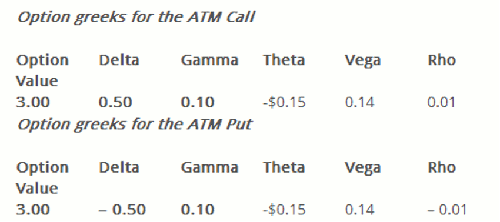Suppose we were to buy 10 straddles. That means we would buy 10 calls and 10 puts. Our total position with greeks would look as follows: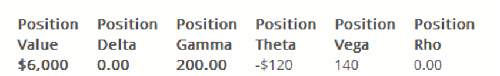Buying ten straddles means we have invested a total of \$6,000 in a trade. While the first two tables showing the individual option greeks were calculated on a per-share basis, the aggregate position shows the value and corresponding greeks calculated on a per-contract basis. Since each option represents 100 shares of stock, the numbers shown appear considerably larger. So, here is the simple scenario that we will cover:

We buy a straddle in stock XYZ, currently trading for \$50 per share. Then, during the course of one day, three events take place in the following order:

Event 1: The stock rises to \$51 per share
Event 2: The stock rises to \$52 per share
Event 3: The stock drops all the way back to \$50 per share

We will now examine two possible reactions that an average trader could have to this series of events.

Reaction 1: Do nothing
If we do nothing, then at the end of the trading day, our stock will be back where it started—at \$50 per share. That means our straddle will be back where it started except that we will have accrued a loss through daily decay (theta) of \$120. Hence, our original \$6,000 position is now worth \$6,000 – \$120 = \$5,880.

Reaction 2: Gamma Scalp
Let’s take a journey through the three events and see what happens to our straddle. Remember that we began with the following aggregate position: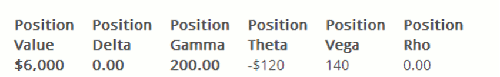We now show what happens as the stock moves per Event 1 from \$50 to \$51.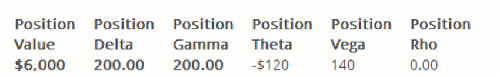For now, we will calculate the effects of the greeks on a discrete one-dollar basis. In real life, the greeks change continuously, but we want to keep things simple.

Let’s now see what happens as the stock moves per Event 2 from \$51 to \$52.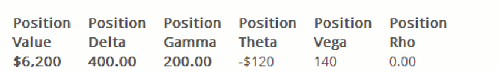Note that since our first dollar move changed our delta from 0 to 200, our position value has increased from \$6,000 to \$6,200. Note further that due to our gamma, our new position delta is now 400, meaning that for the next dollar move we are playing for \$400 per point. At this point, we may decide to implement our first “gamma scalp.” Here’s how it works:

In order to reduce our risk and bring our position back to delta neutral, we sell short 400 shares of XYZ stock at \$52 per share. Our new position with the 400 shares of stock looks as follows: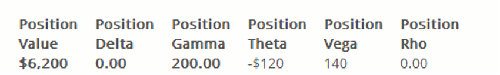We now wait for Event 3—having the stock revert to \$50 per share. Normally, we would end up with the same position that we had at the start of the day. In fact, almost everything remains the same except for our position delta.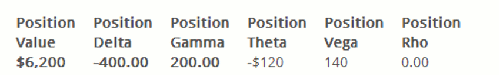With stock back at \$50, we simply buy back our short 400 shares of XYZ. But remember that we sold the shares at \$52 and now get to buy them back for \$50. That pair of transactions on 400 shares of stock spanning two dollars leads to an added \$800 to our position value!

So, at the end of the day, our new position is worth roughly \$6,200 + \$800 = \$7,000. Of course, we now have to take out the \$120 in time decay for a total intra-day gain of \$7,000 – \$120 = \$6,880.

A Few Concluding Remarks
In this simple example, gamma scalping showed that money could be made where it is typically lost. In real life, however, gamma scalping is less of a money-making technique and more of a money-preserving technique. That is, we normally make use of it to help us counter some of the daily decay in long gamma positions. Rarely do we rely on gamma scalping to exceed our daily theta.

Then there are the questions that fit under the “looking for certainty” category, namely: How many shares do I buy or sell? What kind of stock works with this strategy? How far do I let the stock run before gamma scalping? Is there some “magic formula” to help me with my adjustment points?

By Greg Loehr, Owner, OptionABC.com